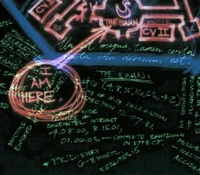## FANDOM

7,386 Pages

There are several equations written as additional notations on the blast door map. At least two of the equations appear to be engineering/physics equations related to magnetics. There is also third equation, a trignometric problem, in the top left corner of the map.

## Basic/Misc.Zoomed-in pic from EW.com, where you can see some of the numbers and dates.
• √16, √64, √225
• Corresponds to 4, 8, 15
• $x 4, y 8, z 15$
• These appear to indicate coordinates, describing some location in reference to three axes.
• Underneath it says "SUBTERRANEAN CONDUIT?"
• "Valenzetti-related" research was conducted on the Island

## Trigonometry

• There are three trigonometric equations in the top left-hand corner of the map.
• $s = 2r \cos 72^\circ$
• $= r\frac{\sqrt5 - 1}{2}$
• $w = 2s \cos 72^\circ = 4r \cos ^2 72^\circ = r[\frac{(\sqrt5 - 1)}{2}]^2$

### Notes/Analysis

• (√5 -1)/2 appears twice in the equation. This number is the golden ratio minus 1 and 1 divided by the golden ratio, which is also known as the golden ratio conjugate. It is also equal to 2 cos 72°.
• cos 72° = -cos 108°.
• A regular pentagon has internal angles 72° and external angles 108°.
• This arrangement can be found in a perfect pentagram. Applying these equations to the pentagram, the total process seeks to find the length of an outer “arm” of the pentagram (w) given the length from one point to another (r).

## B vector, H vector

• There are also two differential equations (calculus) on the map; both are standard engineering/physics equations.
• The B vector equation, on the far right of the map, is magnetic flux density:
$\vec B = {\mu_0 \over {4 \pi}} \int_V \vec \nabla \left ( \vec M \cdot \vec \nabla \left ( {1 \over r} \right ) \right ) dV$.

The equation gives the magnetic field experienced at a given point $r$ due to the presence of a magnetic material with a magnetization given by the magnetization vector $\vec{M}$.

• The H vector equation, in the top left corner of the map, is magnetic field strength:
$\vec H = {\mathcal M \over {\mathcal G_p}} {\partial \over {\partial k}} \vec g$

### Notes/Analysis

• B equation is typically used by physicists to compute the external magnetic field from a magnetic material, i.e. a material which has a diverging Magnetization distribution: $\vec\nabla\cdot\vec M$.
• H fields and B fields are related. B is the magnetic induction field (aka flux density). H is the auxiliary magnetic field. M is the magnetization (magnetic polarization or dipole field).
• $\vec B = \mu_0(\vec H + \vec M)$. Also, $\vec B \ = \mu\vec H$.
• Here $\mu$ is a material dependent property called the permeability.
• M, B, H (and E and D not shown here, but exist in g among other places) need to be specified before these equations have any specific meaning (like saying speed = distance / time). As they stand, the equations just demonstrate an interest in magnets.
• The equations, in their current form, are only useful if the calculator knows the value of magnetization (M), distance (r), and the variables for the g vector.
• k is widely used in electromagnetism equations as representing the wavenumber, so using a k direction in the H equation would be idiosyncratic.
• Gp represents the "operating power gain" of a transmitting antenna (it's not actually a constant) in antenna theory. The H calculation may be to determine the mag-field strength of an antenna.

## Magnitude of B

• There also appear to be two derived values at far right, immediately above "High potential for RVS." Tentatively, they read:
• $B_p => 6 * 10^{-5}$ T
• $B_e => 4 * 10^{-5}$ T

### Notes/Analysis

• The "preposterously high" magnetic field strengths once supposed from the lower resolution map have been clarified by the jigsaw puzzles map, which is clearer. They confirm that the Tesla values are much lower than previously assumed.

## Degrees

A high quality image of the map shows minute markings next to some of the blue lines connecting DHARMA stations. The line from the Staff to the Arrow has written "72°", and the line from the Swan to "C3?" has "56°". Other small markings on the lines are "t-z", "r", and "y". In keeping with trigonometry, it appears to be calculations to work out compass directions to each station.

Community content is available under CC BY-NC-ND unless otherwise noted.